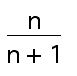# Splitting Fractions in “Two”

2/5 can be written as the sum of two unique unit fractions. For example, 2/5 = 1/3 + 1/15. Try to find two unique unit fractions whose sum is 2/7. What about 2/11? 2/13? Is there a pattern?

(Source: Herb Wills, School Science and Mathematics.)Extension:
Consider all fractions of the formwhere n is a positive integer. Which fractions of this form may be expressed as the sum of two different unit fractions?